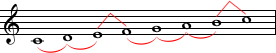# Major Scales

Before we move on, let me introduce the notion of scale. A scale is a set of different notes that have a certain relation together. Depending on that relation, we will define the name of the scale. The first note is called tonic or root. The rest of the notes, called intervals or degrees are assigned by order: second, third, fourth, fifth, sixth, seventh (we could have the eight, which is the same note as the tonic, just an octave higher). Normally, intervals are written in Roman numbers, for example if we see V it refers to the fifth degree, which for instance among the C scale, is G.

A major scale is a succession of 7 notes, one tone away from one another, except between the third and fourth degree, and between the seventh and eighth (exactly the same note as the tonic) which are separated by a semitone. Let’s see that with an example, the C scale:

C D E F G A B

We effectively have one tone (2 frets) between the first and second degrees (C and D), between the second and third (D and E) we have one tone, between the third and fourth (E and F) one semitone (as we remember, there’s always one semitone between E and F), between the fourth and fifth (F and G) there’s one tone, between the fifth and sixth (G and A) one tone, between the sixth and seventh (A and B) we have one tone, and between the seventh and eighth (B and C, which is the tonic again) we have one semitone (as we already know, there’s always one semitone between B and C).

Now, let’s memorize this. The structure of a major scale is always whole-whole-half-whole-whole-whole-half, w-w-h-w-w-w-h as an abbreviation.The pattern of whole and half steps characteristic of a major scale
Illustration by (CC BY-SA 3.0 – original)

That’s it. With this theory we can set up any major scale. Let’s take the G major scale as an example. Well, following the w-w-h-w-w-w-h theory and starting with the G note as it is the tonic, we have:

G A B C D E F♯

Effectively, there is one tone between every note, except between B and C (third and fourth degrees) and F# and G (seventh and eighth). See that I had to use an alteration (a sharp) in order to be bale to set the correct distance between the sixth and seventh, as it has to be one tone. Curiously, this alteration brought the correct distance between the seventh (which we altered) and the eighth, one semitone. Witchcraft? No doubt about that. Let’s take another example, we are going to build the E major scale, but this time, we are going to proceed step by step, following the w-w-h-w-w-w-h rule.

Step 1: write the name of the 7 (8 if we count the octave – in parenthesis below) notes of the scale, without any alteration, just the natural names. Since it is the E major scale, we begin with that very note.

E F G A B C D (E)

Step 2: we correct distances where need be. Between the first and second there must be a full tone, but we know that there’s a semitone between E and F. So we alter F to F# in order to have one tone between the first and second degrees. Now, the scale is:

E F♯ G A B C D

But we need to continue. Now, between the second and third there is one semitone, but we need a full tone in order to follow the rule. We alter G to G#, and it becomes:

E F♯ G♯ A B C D

We are not done yet, let’s continue. Between the third and fourth we need one semitone. Win! Between G# and A there’s already a semitone, so we leave it as is.

We repeat this process until we have checked all the intervals, and if we do this right, we will get:

E F♯ G♯ A B C♯ D♯

We have built the E major scale, that has 4 sharps.

With this system, we can build any major scale we want. If we grab a pen and a piece of paper and we try to write the major scale for the 12 key signatures, we will come up with a sequence of 7 different notes for each major key signature! We will never have 2 identical sequences, each major scale is different on at least one note. Aren’t you impressed?

Exactly as we have written the scale using sharps when needed in order to have the right intervals, we can also use flat notes. The system is the same. Technically, flat notes are used on major scales only if the root or tonic of the scale is flat, except for the F major scale which is always written with flat notes. So let’s sum it up:

• If tonic is not flat and is not F: major scale with sharp notes
• If tonic is F or has a flat: major scale with flat notes

Let’s take an example. We want to build the E♭ major scale. Since the tonic, in this case E♭, is a note that’s altered with a flat, we will build its major scale with flat notes, always respecting the w-w-h-w-w-w-h rule:

E♭ F G A♭ B♭ C D

If we double-check it, the intervals are all correct.

Featured image by Horia Varlan (CC BY 2.0 – original)